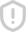### 自动控制原理 第二版习题答案 评分152 第二篇《自动控制原理》习趣解答 么 3)试说明各系统属于哪种控制方式。 解图2-1-1(a)所示系统为按偏差调节的反馈控制系统。设电位计的 输出电压为零时所对应的液面高度为H,当液面的实际高度H≠H0时,电位 计的输出电压不为零,电动机转动带动控制阌门调整输入流量Ω,若H>H, 应诚小输入流量￠,若H<H,则增大输入流量Q,直至=H。水箱为被 控对象,液面高度为被控量,H为给定值,用水流量Q是干扰量。系统的方框 图如图2-1-2所 H 电位计 电动机 控制阀 水箱 浮子 图2-1-2图1-1-1(a)所示系统的方框图 对于图2-1-1(b)所示的系统,当水箱的输入流量Q等于输出流量Q2 时,液面的高度维持不变,设此时的高度为H。当输出流量Q2改变时,液面的 实际高度H发生变化,即H≠H,浮子随液面的高度变化上下移动,并带动杠 杆调节阀门l,改变输入流量Q,直到Q=Q2,使得液面在新的高度上维持不 变。此系统中水箱是被控对象,液面髙度是被控量,希望高度H是给定值,输 出流量Ω3为干扰量。此系统属于按偏差调节的负反馈控制系统。系统的方框 图如图2-1-3所示。 -[子][杆-[门一[水 图2·1·3图2-1-1(b)所示系统的方框图 图2-1-1(所示系统的被控量是水箱内液面的高度H,水箱是被控对象, 希望的高度值为给定值,输出阀门b的变化是干扰量。当输出阀门b改变 时,通过杠杆同时调节输入阀门l,改变输入流量,使得液面的高度维持不变。此 系统属于按干扰补偿的开环控制系统。系统的方框图如图2-1-4所示。 第一章自动控制的一般概念 153 杠杆 阀门 水箱 图2-1-4图2-1-1(c)所示系统的方框图 1-2仓斥大门自动控制系统的原理如图2-1-5所示。试说明自动控制 大门开启和关闭的工作原理,并画出系统的方框图。 四电动白) 开门开关 门 关门开关 图2-15大门开、关控制系统 解当大门处于关闭状态时合上开门开关,电位计电路产生偏差电压,经放 大器放大后,驱动电动机带动绞盘转动,使大门向上提升,与此同时与大门连接 在一起的电位计滑臂上移,直到桥式电路达到平衡,电动机停上转动。反之亦 同。系统的方框图如图2-1-6所示。 桥式电路 放大器 电动机 绞盘 图2-1-6大门开、关控制系统的方框图 1-3位置随动系统的原理如图2-1-7所示。试说明系统的T作原理, 并画出系统的方框图。 R L k ir=常值 图2-1-7位置随动系统 154 第二篇《自动控制原理》习趣解答 解由一对电位计组成误差角检测器,当输入于柄的转角与输出轴的转 角θ.不同时,检测器输出一个与误差角△=0-成正比的电压,经放大器放 大后驱动电动机M转动,经齿轮机构带动负载转动,使Δθ减小,直到△θ=0 时,电机停止。系统的方框图如图2-1-8所示 电位计 佟2-1-8位置殂动系统的方图 第二章 自动控制系统的数宁模型 2-1试求下列函数的拉氏变换式(设t<0时,f(t)=0)。 (1)f(t)=(扌+1)(1+5) (2)f(t)=5(1-cos5t) 05r (3)f(t)=e cos 1ot (4)f( t)=sin 5t+ (5)f(t) 解(1)原函数f(t)可以表示为 f(1)=2+61+5 可得f(1)的拉氏变换式为 F()=2+号+ S (2)原函数f(t)的拉氏变换为 125 S+25s(s+25) 3)根据复位移定理,原函数∫(1)的拉氏变换为 F(5)-(s+05)2+100 (4)原函数f(t)可表示为 f( t)=sin 5 t cos a+cos 5t sin 3 =0 5sin 5 t+0 866Cos 5t 则f(1)的拉氏变换为 F(s)= 25+086680866+25 十 s+25s+25 s+25 (5)原函数f(t)的拉氏变换为 F(s) SS+I T s( Ts+I) 156 第二篇《自动控制原理》习趣解答 2-2求如图2-2-1所示的f(1)的拉氏变换式。 fr 半正弦波 45 45 f(t)图示曲线严 解(a)根据所给图可以确定 f(t)=21(1)+(r-t)1(t-b) 其拉氏变换式为 F(S)=+-2e 〔b)根据所给图可以确定 f(t)=t1(t)-2(t-2)1(t-2)+(t-4)1(t-4) 其拉氏变换式为 2 F(s) (1-2e+e) c)根据所给图可以确定 五 Asin =I 1(1)+ Asin (t-t) I(t-to 其拉氏变换式为 F(S=A (1+e"0) 2-3求下列函数的原函数f(t)。 (1)F(s)= (2)F(s) S+7 (3)F(s) (s+1)(s+3S+2) (4)F( S(s+S+1) 第二章自动控制系统的数学模型 157 (5)F(s) +4s+8 解(1)F(s)的拉氏反变换为 ∫(1)=cos2!-sin2t (t>0) (2)原F(s)可以分解为 ss+5 由此得 f()=÷(1 (>0) (3)原F(s)可以分解为 6 F(s (S+1)(s+2)(s+1)s+1 2 则F(s)的拉氏反变换为 f(t)=61e-5e+5e (t>0) (4)原F(s)可以分解为 F(s) l ++1S 1,3 13 s+ 则F(s)的拉氏反变换为 f(t)=1-e 33.J3 COS SIn t>0) 2 (5)原F(s)可以分解为 F(s)=10s+2 S+2)+4(s+2)+4 则F()的拉氏反变换为 t)=10e 'I cos 2/-sin 21 t>0) 2-4用拉氏变换方法求解下列微分方程。 (1)i(t)+x(t)+x(t)=8(t),x(0)=x(0)=0 (2)i(t)+2x(t)+x()=1(t),x(0)=x(0)=0 (3)(t)+35x(t)+15x(t)=0,x(0) 解(1)方程两边取拉氏变换,得 3 X(s) s+s+1 十 十 158 第二篇《自动控制原理》习趣解答 对上式取拉氏反变换,得 5 x(t)=115e 0866t t>0) (2)方程两边取拉氏变换,得 X(S)= s(s+2s+1)ss+1(s+1) 对上式取拉氏反变换,得 r>0) 3)方程两边取拉氏变换,得 X( x(0)-x(0)+35X()35x(0)+15X(S)=0 0 35s+15 s+3)(s+05) +05 +3 对上式取拉氏反变换,得 x()=04 t>0) 2-5建立下列各机槭系统的动态方程。图2-2-2中x(t)为输入位移 量,y(1)为输出位移量。 x(n x《) r(CI [ y(o) (b) (c) 图2-2-2机械系统 解(a)动态方程为 k d t y) 整理得 f+ ky= kx dt (b)动态方程为 k( r-y d d t d t 第二章自动控制系统的数学模型 159 整理得 d t dy+ ky=kx dt (c)动态方程为 々,d》kxk(x-y)和mdy d t dt =∠ky+k(x-y) 由上述两个方程,得 k kI x di d t 2-6已知圆柱滑阀节流方程为 Q=urdh÷a(P-P2) 流经节流口的流量Q随油口开度e和油口前后压差(P-P2)变化而变化,试 建立其在小扰动条件下的线性方程。工作示意图如图2-2-3所示。 n 图2-2-3滑阀装置示意图 解(1)液压伺服电动机的运动方程 设Q=Q(e,P),其中P=P-P2,设T作点为(的,P),则在工作点附 近有以下关系 人Q=K△c-K△P 其中△e=e-,△P=P·Pa,kQ\,K=·P,由于油液流量△Q 与时间微元dt的乘积等于动力油缸活塞的位移微元dy与活塞面积A及油液 密度p的乘积,即 4pdy=△Qdt 于是有如下关系式 AP=T(K. Ae-Ap

...展开详情举报收藏分享

### 评论下载该资源后可以进行评论共1条

2012-12-27《自动控制原理》课后习题答案 立即下载《自动控制原理》胡寿松 第四版课后答案 立即下载longying9080